In organic chemistry, we need to understand how the conformation works. This is because organic compounds have a three-dimensional structure, not a planar one. By studying stereochemistry, you can predict whether a chemical reaction will proceed or not.

Also, even the same molecule has different conformations. The energies are different depending on where the substituents are placed.

One of the first things you will learn in stereochemistry is the conformation of alkanes. In the stereochemistry of alkanes, we use the Newman projection. The Newman projection describes the state of a molecule by focusing on the rotation of its axes.

By using Newman projection, we can understand the conformation of alkanes. In this section, we will explain the 3D structure of alkanes, which is the first step in stereochemistry.

## Relationship Between the Fischer and Newman Projection

When writing the structural formula of an organic compound, there are several methods available. Among them, the Fischer projection is often used.

Fischer projection is used to describe the structure of a molecule in two dimensions, even in the presence of chiral carbons.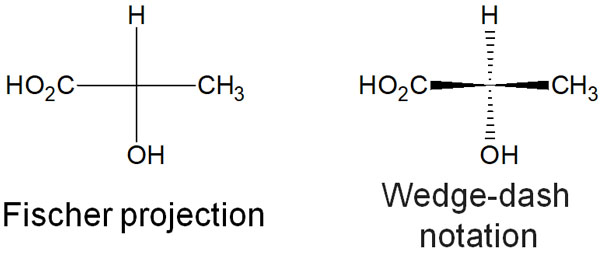When there is a chiral center, a technique often used is dashed-wedge notation. Although the molecule is described in the plane, a dashed line means that the substituents are in the back. In a wedge shape, the substituents are in the front. On the other hand, the Fischer projection describes all bonds in a plane.

-Fischer Projection Does Not Show the Conformation

However, the Fisher projection has the disadvantage that the conformation of the molecule cannot be determined at all. For this reason, the Newman projection is often used when considering stereochemistry.

The Newman projection is used to show the positions of substituents in the same molecule. For example, we have the following.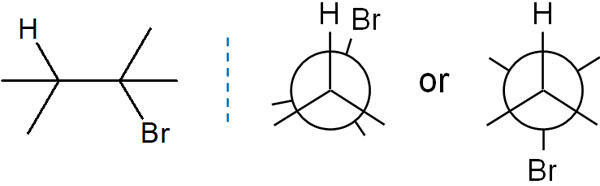Even if you can’t understand it in the Fisher projection, you will be able to understand the conformation of the molecule in Newman projection.

### Considering the Alkane Conformation in the Newman Projection

It is impossible to understand how alkanes work in stereochemistry without using the Newman projection. So how can we use the Newman projection? First, let’s think of the Fischer projection in three dimensions.

All molecules are three-dimensional. The Newman projection is one of the methods to think of molecular structures in three dimensions. Another characteristic of Newman projection is that we look at the molecule from the side.

For example, the Newman projection for ethane looks like the following.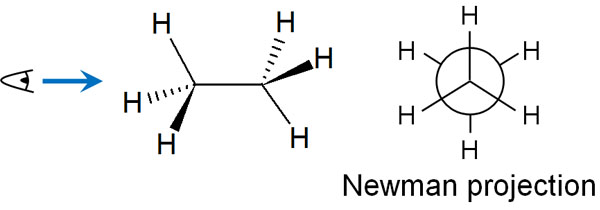The Newman projection is used to check what substituents exist around the C-C bond (bond between carbons), which is the center of the molecule.

At the center of the Newman projection, two carbon atoms overlap. The Newman projection is what you see from the side of the axis. By learning this method, you will be able to understand how the energies differ depending on the conformation of the molecule.

## For Single-Bond (σ-Bond), the Axis Rotates and the Dihedral Angle Is Important

Why do we need to consider the Newman projection? This is because, in a single bond (σ bond), the axis is free to rotate. For example, in ethane, the axis of the C-C bond rotates as shown below.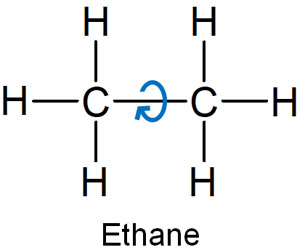In a double or triple bond, there is no rotation of the axis. Therefore, we don’t write Newman projection for compounds with double bonds. The Newman projection is used to think about the conformation of a compound due to the rotation of the axis, and there is no point in using the Newman projection for double or triple bonds that do not have rotation.

For single bonds where the axis rotates, we need to consider the dihedral angle. Looking at the molecule from the side of the axis, as in the Newman projection, can cause strain due to the dihedral angle.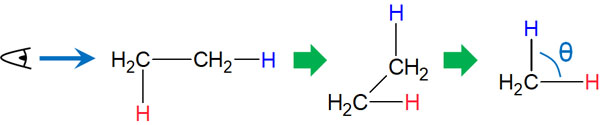The ideal dihedral angle (θ: theta) is 60°. In some cases, however, the dihedral angle may be 0°. Since the axis is free to rotate, the dihedral angle will vary depending on the situation. The Newman projection can help us to understand the dihedral angle strain.

Think of the Newman projection as a tool to understand the dihedral angular strain.

### Staggered Conformations Are More Stable Than Eclipsed Conformations

What kind of strain is produced at a dihedral angle when the axis of a molecule rotates? Let’s consider the example of ethane.

First, let’s consider the case that the dihedral angle (θ) becomes 60° or 0° due to the rotation of the C-C bond in ethane. In this case, when the dihedral angle is 60°, it is called a staggered conformation. On the other hand, when the dihedral angle is 0°, it is called an eclipsed conformation.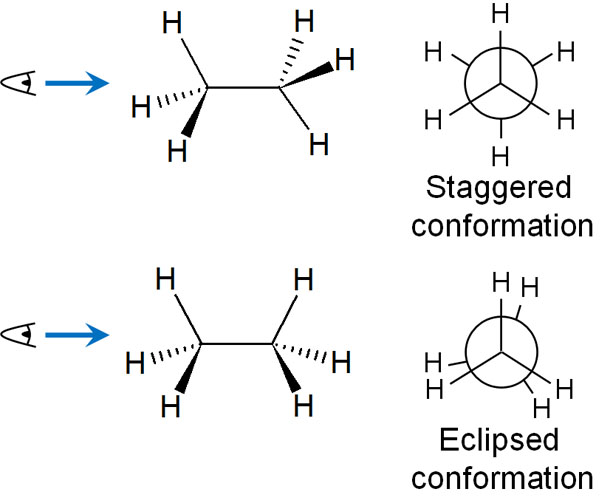The rotation of the axis can take two forms: a staggered conformation and an eclipsed conformation. By the way, as for the eclipsed conformation, it actually overlaps perfectly. However, if you write them overlapping in the Newman projection, you cannot see the substituents bonded to the carbon atoms behind, so it is common to write them slightly shifted like this.

In this case, which is more stable, the staggered conformation or the eclipsed conformation? Molecules are more stable in the staggered conformation than in the eclipsed conformation.

### Rotation of the Axis Causes Differences in Potential Energy

Repulsion of electrons is involved in the reason for instability in the eclipsed conformation. By sharing electrons, molecules form covalent bonds. In this case, electrons with a negative charge repel each other.

In the eclipsed conformation, the electrons involved in the bond repel each other, making it unstable. If the dihedral angle is 0°, the energy increases due to the strain.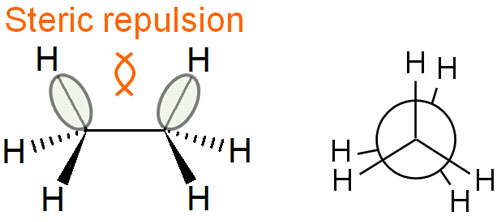Although the axis can rotate freely, the energy in the molecule can be higher or lower depending on whether the dihedral angle is 0° or 60°.

When the dihedral angle is 0°, the potential energy is high. On the other hand, when the dihedral angle is 60°, the potential energy is low. The energy diagram is as follows.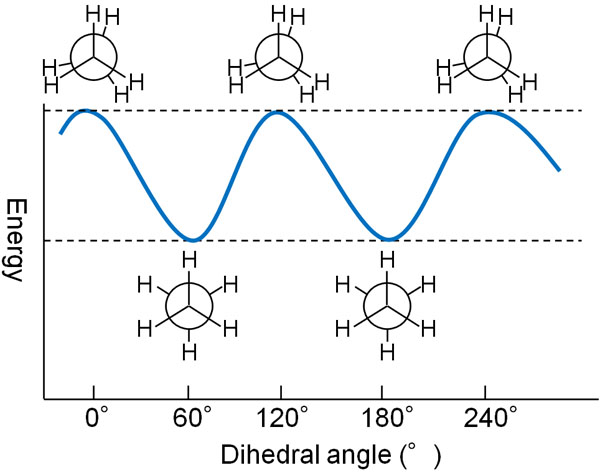If you represent the dihedral angle in the Newman projection, you should understand that the potential energy varies with the angle, as shown here.

## Butane Has Different Stable Structures in the Anti and Gauche Forms

What about compounds that have more substituents than ethane? Butane is frequently used as an example of this.

Propane has the same shape potential energy diagram as ethane. Therefore, both ethane and propane have the same energy difference due to their conformation. Butane, on the other hand, has a more complicated stable structure.

When writing the structure of butane in the Newman projection, we can come up with the following two ways in the staggered conformation.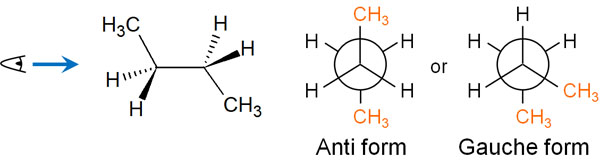Among the staggered conformation, we can write a structure where the methyl group on one side is at 180°. This conformation is called anti form.

On the other hand, we can write a Newman projection where the methyl group is at the 60° position. This conformation is called the gauche form.

Is there any difference in the potential energy between the anti-form and the gauche-form? For this, the potential energy is higher in the gauche form. The reason for this is that the methyl groups are close together, so they repel each other sterically.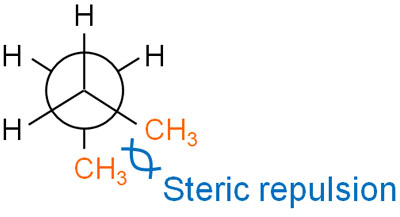When we consider stability in stereochemistry, we have to check for steric hindrance.

In the anti forms, the methyl groups are at 180°, so there is no steric hindrance. On the other hand, in the gauche form, the methyl groups are at 60° to each other and are sterically repelled. Therefore, the strain in the gauche form is higher than in the antique form, and the energy is higher. The anti form is more stable than the gauche form.

### Staggered and Eclipsed Have Different Energies Due to Substituents

The same is true for the eclipsed conformation as well as the staggered conformation configurations. In the same way that the energy of the staggered conformation differs depending on the position of the methyl group, the energy state of the eclipsed conformation differs depending on the position of the substituent.

In the eclipsed conformation, we can come up with the following two conformations.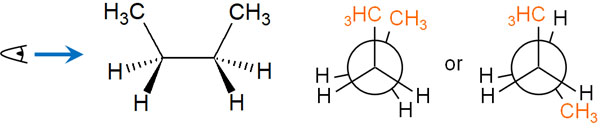When considering steric hindrance, a form with overlapping methyl groups has a very high energy state and is unstable. Methyl groups are much larger in shape than hydrogen atoms. When the large substituents are in close proximity to each other, they have high energy.

On the other hand, when the methyl groups are separated from each other in the eclipsed conformation, the energy state is lower. Of course, since the bonds are overlapping, the energy state is still high and unstable. However, the energy state is more stable than the state where the methyl groups overlap each other.

### Potential Energy Diagram of Alkanes That Varies with the Position of the Methyl Group

When we consider the Newman projection for butane, what does the potential energy look like as the C-C axis (single bond of carbon) rotates? The energy diagram of the stable (or unstable) state is shown below.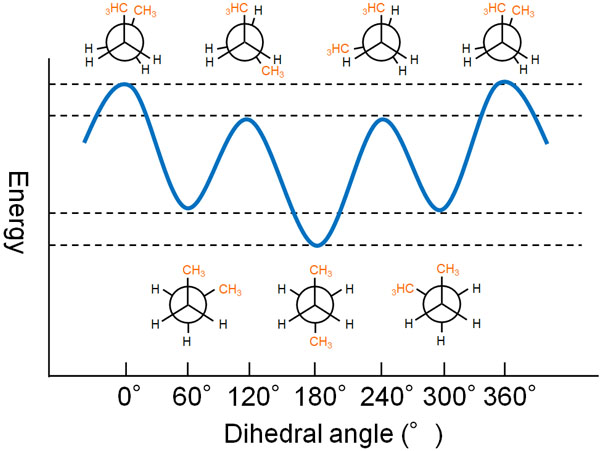The potential energy of butane is different depending on the type of conformation.

In the staggered conformation, the energy is lower and more stable. However, as shown in the above figure, when the methyl group is in the gauche form, the energy is slightly higher. On the other hand, the energy is the lowest and most stable when the methyl group is in the anti form because there is no steric hindrance.

In the eclipsed conformation, the energy is most unstable where the methyl groups overlap. In contrast, when the methyl groups do not overlap, the energy is slightly lower.

## Understanding Energy in Stereochemistry

In most cases, in organic chemistry, the structural formula is written in a planar form. For example, by using the Fischer projection, we can write the structural formula in a plane, even if there are stereoisomers.

However, all molecules are three-dimensional. In addition, the axis of a single bond can rotate freely, which is not the case with double or triple bonds. Therefore, the energy possessed by the molecule differs depending on the conformation of the molecule. In such cases, the Newman projection is used to make it easier to understand.

The first thing to consider in the Newman projection is the eclipsed and staggered conformations. The ideal dihedral angle is 60°; any deviation from this angle will result in high energy and instability.

Not only that but if the molecule has more substituents, understand that there are anti forms and gauche forms. Once you learn that a molecule has different energies, you will be able to understand more details about the shape of the molecule. You will also be able to guess how easy it is for synthetic reactions to occur.